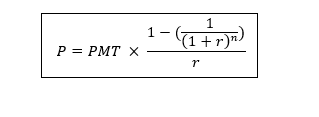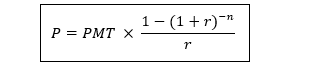# Annuity Table

• Updated on

## What is an annuity table?

An annuity table is a financial tool that is used to calculate the present value of an annuity. It is also known as the present value table. The present value interest factor (on an annuity) is calculated in the table.

Present value refers to the current value of a future income stream with a given rate of return. The present value of an annuity contract is not equal to the accumulated value of a contract because of the time value of money. Since the payment will be received on a future date, the actual worth of the contract is less at the present date because of the time value of money theory.

The present value interest factor of an annuity (PVIFA) is multiplied with the recurring payment amount to achieve the present value of a contract.

## Summary

• An annuity table is a financial tool that is used to calculate the present value of an annuity.
• It is also known as the present value table.
• The present value of an annuity contract is not the accumulated value of a contract because of the time value of the money.

## What is the time value of money?

Time value of money projects that one dollar today will have more worth at any time on a future date. The theory is validated with the statement that every dollar has the potential to earn as it can be invested and returns can be enjoyed.

For example, if an individual invests \$2000 today in a high-yield bond which will provide a yield of 1 per cent annually, then after one year the individual will have \$2020.Image Source: © Marchmeena | Megapixl.com

## Who uses the annuity table?

Annuity tables are used by actuaries, accountants, insurance personnel, or financial investors, who consider the amount invested today into an annuity and the time of investment with the aim of determining the amount due to the annuity buyer.

Lottery winners also utilise the annuity table to determine whether to take money in the form of an annuity or as a lump sum amount. If the present value of the annuity is greater than the lump sum amount, then payment should be received in the annuity.

Retirement planning can become easy with the employment of annuity tables, as the financial plans can be tailored as per the current financial status. Moreover, the investor can determine the real value of their investment at any time in future.

It can also be deployed while managing the budget, as annuity tables empower an individual to manage their finances as per their present savings or investment.## What is the working of an annuity table?

The annuity table provides a value based on the time and discount rate, which is the same as the interest rate. Multiplying the discount rate with the annuity payment results in the present value of an annuity.

The annuity table works on the principle of time value of money which states that receiving \$10,000 on the present date is better than receiving \$1000 for 10 years, as the amount can be reinvested and profits can be earned. The invested \$10,000 would have more value in future because of the interest received on the amount.

## What are the benefits of an annuity table?

The annuity table help in avoiding the complex calculations of the present value. Present value is calculated by multiplying values visible on the PFA table with the annuity amount.  Therefore, the annuity table allows ease of use.

## How to calculate the present value of an annuity?

To calculate the present value of an annuity, the interest rate and time should be known beforehand.

It can be determined by using two formulas:ORHere PMT = annuity amount

r = rate of interest

n = Number of periods

## How to use an annuity table?

Firstly, an individual must know the date at which payment will be received, that is the beginning of the year or the end of the year. Generally, the payment is received at the end of a period. When payment has to be received at the beginning of a year, it is remarked as an annuity due. It applies to the expense which occurs during the first month such as car lease or rent.

For using the annuity table, the user only needs to know the payment amount, discount rate (rate of interest) and the number of payments or years of payment. This information is generally available in the contact.

By using the annuity table, the calculations can be avoided. On the y – axis, the number of payments and on the x – axis the discount rate is mentioned. As per the individual’s annuity, find both values and find the cell where they intersect. Afterwards, the value in the cell is multiplied by the amount of annuity received, and the present value of the annuity will be ascertained.

## What are the limitations of the annuity table?

The values in the annuity tables are not precise in comparison to the financial software programmes or the manual calculations. The tables contain a limited set of payment and interest rate values.

Moreover, the values in the tables are within a decimal place and might be rounded off.

Lastly, the table cannot be used when the interest rate and payments are not fixed. For example, an individual receives a payment of \$1000 for the first 5 years, \$3500 for the next 2 years and \$1000 for the next 3 years. In such a case annuity table won’t be helpful.

## What other methods can be adapted to calculate the present value of an annuity?

There are different methods for calculating the present value of an annuity, and each method requires a different skillset and mathematical calculations. However, these methods will give a more precise and accurate value in comparison to the annuity table.

• Annuity calculator